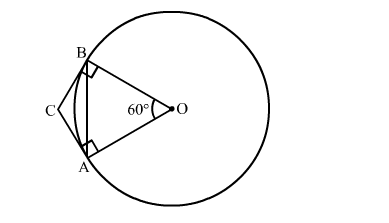# If a chord AB subtends an angle of 60∘ at the centre of a circle,`
Question:

If a chord AB subtends an angle of 60 at the centre of a circle, then the angle between the tangents to the circle drawn from A and B isl to

(a) 30
(b) 60
(c) 90
(d) 120

Solution:We know that the radius and tangent are perperpendular at their point of contact
∵∠OBC = ∠OAC = 90